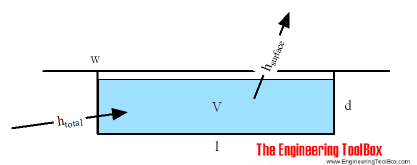4. Heating System

Pool Heaters can increase the comfort level of your pool even if the environment naturally dictates a pool a little cooler than you would like.

The total required heat to increase and maintain the temperature in an outdoor pool can be calculated as

htotal = hsurface + hheat-up                                              (1)

where

htotal = total heat load (btu/hr, W)

hsurface = heat loss from pool through the surface – mainly evaporation of water (btu/hr, W)

hheat-up = heat load required to increase the pool temperature (btu/hr, W)The heat-up load depends on the volume of the pool. The volume can be calculated as:

V = 7.5 l w d                                             (2)

where

V = volume (Gal)

l = length (ft)

w = width (ft)

d = depth (ft)

The heat-up load can be calculated as

hheat-up = 8.34 V dTw / dt                                             (3)

where

dTw = difference between initial temperature and the final temperature of the water in the pool (oF)

dt = heat pick-up time (hr)

Swimming Pool Heat-Up Load or Heat-Up Time Calculator – Imperial Units

Add the required data for your swimming pool below. Note that you must use either “Heat-Up Load” or “Heat Pick-up Time” in the calculator.

Note that heat loss during heat-up is not included in the calculation.

Surface Heat Loss due to Temperature Difference

The heat load required to replace the surface heat loss due to the temperature difference between the pool surface and the ambient air can be expressed as

hsurface = ks dTaw A                                           (4)

where

ks = surface heat loss factor – for sheltered positions with average wind velocity 2 to 5 (mph), the surface heat loss factor is in the range 4 to 7 (Btu/hr ft2 oF)

dTaw = temperature difference between the air and surface water in the pool (oF)

A = surface area of the pool (ft2)

Note that the major part of the heat loss from a swimming pool surface is a result of evaporation of water from the surface.

Example – Pool Heating

A pool with dimensions length 30 ft, width 20 ft and dept 6 ft is heated from 50oF to 75oF. The volume in the pool (in gallons) can be calculated as

V = (30 ft) (20 ft) (6 ft) (7.5 gal/ft3)

= 27000 (gal)

The heat-up heat required to heat the pool up in 24 hours can be calculated as

hheat-up = (27000 gal) (8.34 lbs/gal) ((75 oF) – (50 oF)) (1.0 Btu/lb oF) / (24 hr)

= 234562 Btu/hr

• 1 Btu/hr = 2.931×10-4 kW = 0.252 kcal/hr

Note that this value is without the increased heat loss through the surface due to the increased temperature difference and increased evaporation when the temperature increases.

If the ambient temperature is 65 oF and the wind conditions is modereate, the heat loss through the surface due to temperature difference (when the pool is heated up) can be calculated as

hsurface = (5 Btu/hr ft2 oF) ((75 oF) – (65 oF)) (30 ft) (20 ft)

= 30000 (Btu/hr)

Heat-up Load in SI-units

The heat-up load in SI-units can be calculated as

hheat-up =  ρ cp l w d dT / dt                                                  (5)

where

hheat-up = heat flow rate required (kW, kJ/s)

ρ = 1000 – density of water (kg/m3)

cp = specific heat water (4.2 kJ/kgoC)

l = length of swimming pool (m)

w = width of swimming pool (m)

d = dept of swimming pool (m)

dT = difference between initial and final temperature (oC)

dt = heat up time (sec)

Example – Heat-up Load in SI-units

The heat-up load for a swimming pool with dimensions 12 m x 6 m x 1.5 m, heated from 10oC to 20oC in 10 hours, can be calculated as

hheat-up =  (1000 kg/m3) (4.2 kJ/kgoC) (12 m) (6 m) (1.5 m) ((20 oC) – (10 oC)) / ((10 hours) (3600 sec/hours))

= 126 (kW)

Swimming Pool Heat-Up Load or Heat-Up Time Calculator – SI Units

Add the required data for your swimming pool below. Note that you must use either “Heat-Up Load” or “Heat Pick-up Time” in the calculator.

Note that heat loss during heat-up is not included in the calculation.